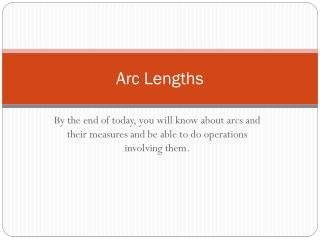DownloadDownload PresentationArc Lengths

# Arc Lengths

Download Presentation## Arc Lengths

- - - - - - - - - - - - - - - - - - - - - - - - - - - E N D - - - - - - - - - - - - - - - - - - - - - - - - - - -
##### Presentation Transcript

1. Arc Lengths By the end of today, you will know about arcs and their measures and be able to do operations involving them.

2. Arc Length • Arc length is a part of the circumference of a circle and is found by using a proportion. arc length = arc measure circumference 360

3. Example • In Circle P, find the length of arc AB

4. Central Angle • A central angle of a circle is an angle whose vertex is the center of the circle. • When we say the measure of an arc, we are referring to the degree measure. • When we say the length of the arc, we are referring to the portion of the circumference the arc covers. Measure of an Arc Length of an Arc

5. Notation • When naming an arc, for instance arc AB, we use the notation • When talking about the degree measure of an arc, we use the notation

6. Minor Arc • The measure of a minor arc is the measure of its centralangle. • The measure of the entire circle is 360o. The measure of a major arc is the difference between 360o and the measure of the related minor arc. Major Arc

7. Semicircle • A semicircle is an arc the endpoints that are the endpoints of a diameter.

8. Arc Addition Postulate • The measure of an arc formed by two adjacent arcs is the sum of the measures of the two arcs

9. Congruent Circles • Two circles are congruent circles if they have the same radius. • Two arcs are congruent arcs if the have the same measure and they are arcs of the same circle or of congruent circles. Congruent Arcs

10. Tell whether the red arcs are congruent.

11. Assignment • p.661: 3-14 • p.751: 15-23